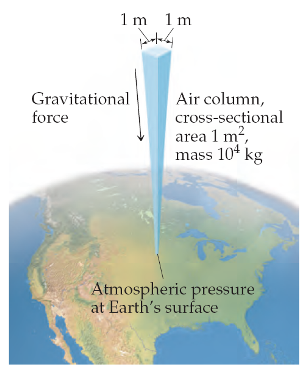# Problem: Assume the top of your head has a surface area of 25 cm×25 cm.Calculating atmospheric pressure.How many newtons of force push on your head at sea level?

###### FREE Expert Solution

$\overline{){\mathbf{Pressure}}{\mathbf{=}}\frac{\mathbf{Force}}{\mathbf{Area}}}$

91% (59 ratings)###### Problem Details

Assume the top of your head has a surface area of 25 cm×25 cm.Calculating atmospheric pressure.

How many newtons of force push on your head at sea level?